1. /
2. CBSE
3. /
4. Class 07
5. /
6. Science
7. /
8. NCERT solutions for Class...

# NCERT solutions for Class 7 Science Motion and time

NCERT solutions for Science Motion and time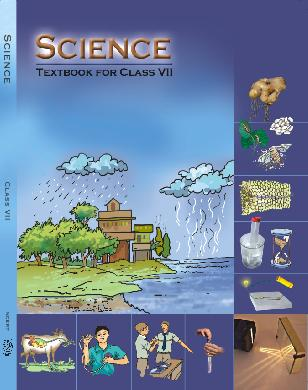## NCERT Class 7 Social Science Chapter wise Solutions

1. Nutrition in Plants
2. Nutrition in Animals
3. Fibre To Fabric
4. Heat
5. Acids Bases And Salts
6. Physical And Chemical Changes
7. Climate
8. Winds Storms And Cyclones
9. Soil
10. Respiration In Organisms
11. Transportation in Animals And Plants
12. Reproduction In Plants
13. Motion And Time
14. Electric Current And Its Effects
15. Light
16. Water
17. Forests: Our Lifeline
18. Waste Water Story

## NCERT solutions for Class 7 Science Motion and time

###### Question 1.Classify the following as motion along a straight line, circular or oscillatory motion.

(i)Motion of your hands while running.

(ii)Motion of a horse pulling a cart on a straight road.

(iii)Motion of a child in merry-go –round.

(iv)Motion of a child on a see-saw.

(v)Motion of the hammer of an electric bell.

(vi)Motion of a train on straight bridge.

Classification of motion

(i)Oscillatory motion

(ii)Straight line

(iii)Circular motion

(iv)Oscillatory motion

(v)Oscillatory motion

(vi)Straight line.

###### Question 2.Which of the following are not correct?

(i)The basic unit of time is second.

(ii)Every object moves with a constant speed.

(iii)Distance between two cities is measured in kilometers.

(iv)The time period of a given pendulum is not constant.

(v)The speed of a train is expressed in m/h.

(ii), (v).

NCERT solutions for Class 7 Science Motion and time

###### Question 3.A simple pendulum takes 32 s to complete 20 oscillations. What is the time-period fo the pendulum.

Time taken for 20 oscillation = 32 s

Time taken for 1 oscillation = 32s/20.

Time period = 1.6 s

###### Question 4.The distance between two stations is 240 km. A train takes 4 hours to cover this distance. Calculate the speed of the train.

Distance = 240 km

Time = 4 h

Speed = distance/time

= 240 km/4 h

= 60 km/h

NCERT solutions for Class 7 Science Motion and time

###### Question 5.The odometer of a car reads 57,321.0 km when the clock shows the time 8.30 AM. The odometer reading was changed to 57,336.0 km. calculate the speed of the car in

km/min during this time. Express the speed in km/h also.

Odometer reading at 8.30 AM = 57321.0 km

Odometer reading at 8.50 AM = 57336.0 km

Distance travelled = 15 km

Time taken = 20 min.

Speed = distance / time

= 15 km/20 min.

= 0.75 km/h = 0.75 x 60 = 45 km/h.

###### Question 6.Salma takes 15 minutes from her house to reach her school on bicycle. If the bicycle has a speed of 2 m/s, calculate the distance between her house and the school.

Speed = 2 m/s

Time = 15 min = 15 x 60 s = 900 s.

Distance travelled = speed x time

2 x 900 s = 1800 m = 1.8 km.

NCERT solutions for Class 7 Science Motion and time

###### Question 7.Show the shape of the distance-time graph for the motion in the following cases:

(i)A car moving with a constant speed.

(ii)A car parked on a side road.

(i)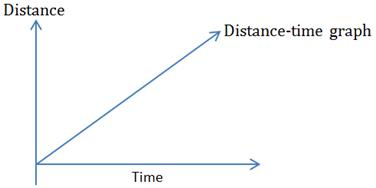###### Question 8.Which of the following relations is correct?

(i)Speed = Distance x Time.

(ii)Speed = {tex}\frac{{Distance}}{{Time}}{/tex}

(iii)Speed = {tex}\frac{{Time}}{{Distance}}{/tex}

(iv)Speed = {tex}\frac{1}{{Distance\;\,\, \times \;Time}}{/tex}

(ii) Speed = {tex}\frac{{Distance}}{{Time}}{/tex}

NCERT solutions for Class 7 Science Motion and time

(i)Km/min

(ii)m/min

(iii)km/h

(iv)m/s

(iv) m/s.

###### Question 10. A car moves with a speed of 40 km/h for 15 minutes and then with a speed of

60 km/h for the next 15 minutes. The total distance covered by the car is :

(i)100 km

(ii)25 km

(iii)15 km

(iv)10 km

(ii) 25 km

NCERT solutions for Class 7 Science Motion and time

###### Question 11.Suppose the two photograph, shown in Fig. 13.1 and Fig.13.2, had been taken at an interval of 10 seconds. If a distance of 100 meters is shown by 1 cm in these photograph, calculate the speed of the blue car.

Speed = 100 m/10s = 10 m/s

###### Question 12.Fig. 13.5 shows the distance-time graph for the motion of two vehicles A and B. which is one of them moving faster?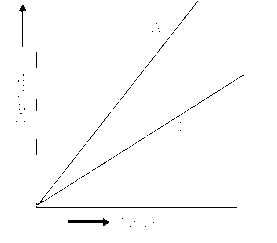NCERT solutions for Class 7 Science Motion and time

###### Question 13. Which of the following distance time-graph a truck moving with speed which is not constant.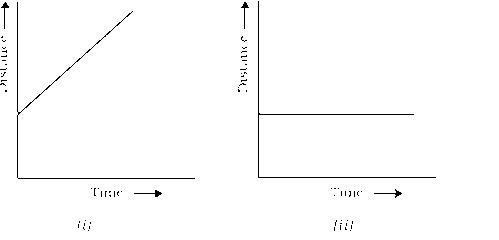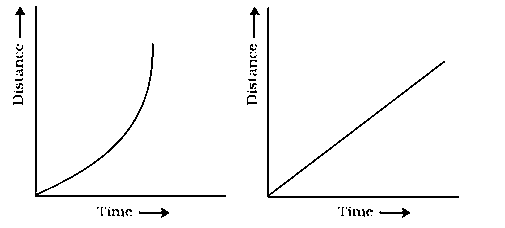## NCERT solutions for Class 7 Science

NCERT Solutions Class 7 Science PDF (Download) Free from myCBSEguide app and myCBSEguide website. Ncert solution class 7 Science includes text book solutions for CBSE Class 7 Science have total 18 chapters. 7 Science NCERT Solutions in PDF for free Download on our website. Ncert Science class 7 solutions PDF and Social Science ncert class 7 PDF solutions with latest modifications and as per the latest CBSE syllabus are only available in myCBSEguide.

## CBSE app for Class 7

To download NCERT Solutions for class 7 Social Science, Computer Science, Hindi ,English, Maths Science do check myCBSEguide app or website. myCBSEguide provides sample papers with solution, test papers for chapter-wise practice, NCERT solutions, NCERT Exemplar solutions, quick revision notes for ready reference, CBSE guess papers and CBSE important question papers. Sample Paper all are made available through the best app for CBSE students and myCBSEguide website.### Test Generator

Create question paper PDF and online tests with your own name & logo in minutes.### myCBSEguide

Question Bank, Mock Tests, Exam Papers, NCERT Solutions, Sample Papers, Notes